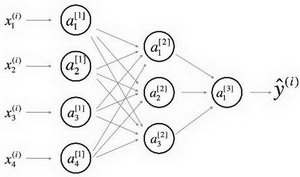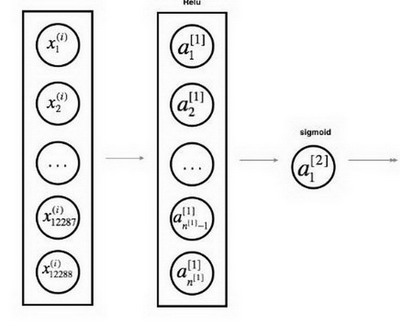# 深度学习符号

## 常用的定义

• 原版符号定义中，$x^{(i)}$$x_i$ 存在混用的情况，请注意识别

### 数据标记与上下标

• 上标 $^{(i)}$ 代表第 $i$ 个训练样本

• 上标 $^{[l]}$ 代表第 $l$

• $m$ 数据集的样本数

• 下标 $_x$ 输入数据

• 下标 $_y$ 输出数据

• $n_x$ 输入大小

• $n_y$ 输出大小 (或者类别数)

• $n_h^{[l]}$$l$ 层的隐藏单元数

• $L$ 神经网络的层数

• 在循环中

• $n_x = n_h^{}$
• $n_y = n_h^{[L + 1]}$

### 神经网络模型

• $X \in \mathbb{R}^{n_x \times m}$ 代表输入的矩阵

• $x^{(i)} \in \mathbb{R}^{n_x}$ 代表第 $i$ 个样本的列向量

• $Y \in \mathbb{R}^{n_y \times m}$ 是标记矩阵

• $y^{(i)} \in \mathbb{R}^{n_y}$ 是第 $i$样本的输出标签

• $W^{[l]} \in \mathbb{R}^{l \times (l-1)}$ 代表第 $[l]$ 层的权重矩阵

• $b^{[l]} \in \mathbb{R}^{l}$ 代表第 $[l]$ 层的偏差矩阵

• $\hat{y} \in \mathbb{R}^{n_y}$ 是预测输出向量

• 也可以用 $a^{[L]}$ 表示

#### 正向传播方程示例

• $a = g^{[l]}(W_x x^{(i)}_ + b_1) = g^{[l]}(z_1)$

• 其中， $g^{[l]}$ 代表第 $l$ 层的激活函数
• $\hat{y} = softmax(W_h h + b_2)$

#### 通用激活公式

• $a_j^{[l]} = g^{[l]}(z_j^{[l]}) = g^{[l]}(\sum_k w_{jk}^{[l]}a_k^{[l-1]} + b_j^{[l]})$

• $j$ 当前层的维度
• $k$ 上一层的维度

#### 损失函数

• $J(x, W, b, y)$ 或者 $J(\hat{y}, y)$

• 常见损失函数示例

• $J_{CE}(\hat{y}, y) = -\sum_{i=0}^m y^{(i)}log\hat{y}^{(i)}$
• $J_1(\hat{y}, y) = -\sum_{i=0}^m |y^{(i)} - \hat{y}^{(i)}|$

## 深度学习图示

• 节点：代表输入、激活或者输出
• 边：代表权重或者误差

### 详细的网络### 简化网络